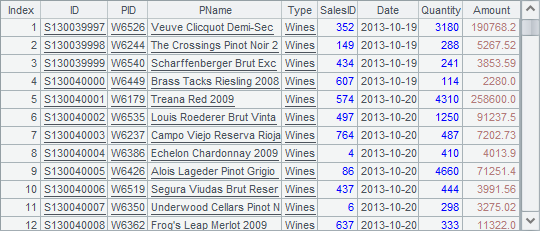# 组表的访问

 A B 1 =file("D:/file/dw/employees.ctx") 2 =A1.open() =A2.cursor().fetch() 3 =A2.attach(stable) =A3.cursor().fetch() 4 =A3.cursor(EID,Dept,Gender,Name,OCount,OAmount).fetch()

A2中，使用无参数的create函数打开组表的基表，在A3中，仅指定附表的名称，用T.attach函数打开实表。在B2中查询出组表基表中数据如下：B3中查询出实表stable中数据如下：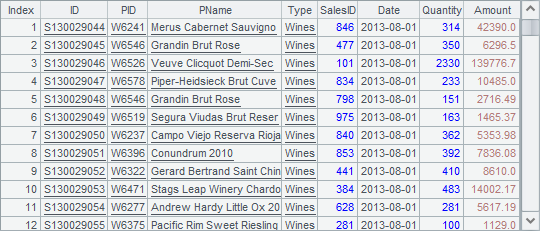A B 1 =file("D:/file/dw/employees.ctx") 2 =A1.open() =A2.attach(stable) 3 =A2.cursor(EID,Name,Dept) =A3.fetch() 4 =A2.cursor(;right(Name,1)=="e") =A4.fetch() 5 =B2.import(EID,Name,OCount,OAmount;OAmount>5000)

A3中仅从组表的基表中取出部分字段，B3fetch获得的结果如下：A4中设置了查询姓名最后一个字母为e的员工数据，B4中获得的结果如下：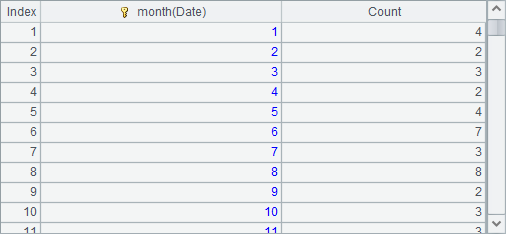A B 1 =file("D:/file/dw/employees.ctx") =A1.open() 2 =B1.attach(stable) 3 =file("D:/file/dw/orders.ctx") =A3.open() 4 =B3.attach(otable) 5 =A2.cursor(;;1:3).fetch() =A2.cursor(;;2:3).fetch() 6 =A4.cursor(;;1:3).fetch() =A4.cursor(;;2:3).fetch()

A2中从组表文件employees.ctx中取出销售员实表stableA5B5中，从销售员实表中分段取数，都分为3段，但分别取出第1段和第2段，结果分别如下：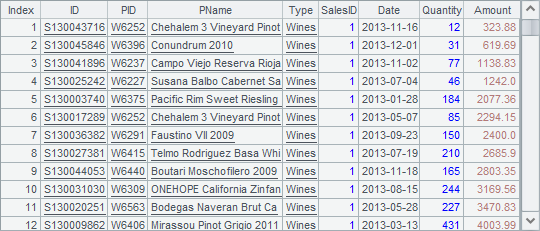A4打开组表文件orders.ctx中的订单实表otableA6B6中，从订单实表中分段取数，同样分为3段，并分别取出第1段和第2段，结果分别如下：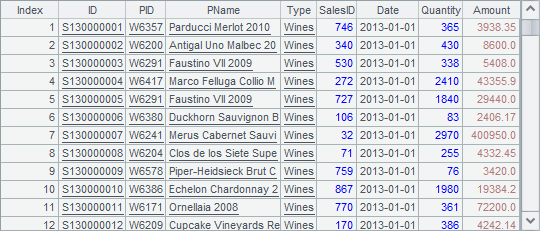A B 1 =file("D:/file/dw/employees.ctx") =file("D:/file/dw/orders.ctx") 2 =A1.open() =B1.open() 3 =A2.attach(stable) =B2.attach(otable) 4 =A3.cursor@m(;;3) =B3.cursor(;;A4) 5 =joinx(A4:s,EID;B4:o,EID) =A5.fetch() 6 =A3.cursor@m(;;3) =B3.cursor(;;A6) 7 =joinx(A6:s,EID;B6:o,EID) =A7.groups(s.EID:EID;count(~):Count, sum(o.Amount):Sum)

A3B3中，分别在两个不同的组表中，读出实表stable和实表otable。在A4中，用函数T.cursor@m(;;n) 将实表stable分为n段生成多路游标，在B4中用实表otable根据A4中的多路游标同步分段。执行同步分段后，在A5中将A4B4中的游标用joinx函数将同步的多路游标连接起来，在B5中可以看到连接的结果：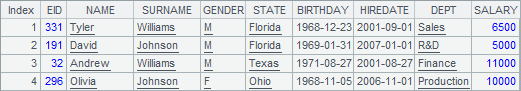B7中，重新生成多路游标的连接，并计算出每个销售员的订单总数和销售总数，结果如下：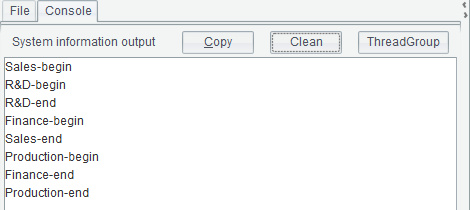A B 1 =file("D:/file/dw/employees.ctx") =A1.open() 2 =B1.import(;) 3 =now() 4 =B1.cursor(;[8136,11247,83372,264,8223,826, 32758,1341,6255,1983].contain(EID)) =A4.fetch() 5 =interval@ms(B3, now()) 6 >B1.index(idx, [8136,11247,83372,264,8223, 826,32758,1341,6255,1983].contain(EID);EID) =now() 7 =B1.icursor(;[8136,11247,83372,264,8223, 826,32758,1341,6255,1983].contain(EID),idx) =A7.fetch() 8 =interval@ms(B6, now())

12行打开组表employees.ctx中的基表员工实表。在第4行，直接从实表中查询10条记录，B4中得到结果如下：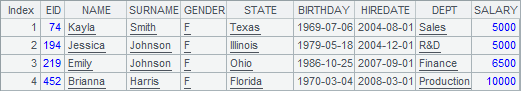A6中，用函数T.index(I, w; C,…) 为实表生成索引，A6中将索引命名为idx，并根据键EID生成。执行后，索引文件将自动生成，文件名自动处理。在A7中使用T.icursor(C,…; w, I) 函数查询时，可以根据索引文件来执行。B7中得到的结果和B4中是相同的。调用T.index(I, w; C,…) 函数时如果未设字段C,…，则会删除实表T的索引I，如果无参数I，则会删除实表T的所有索引。

B5B8中分别计算出两种情况下查询10条记录的耗时如下：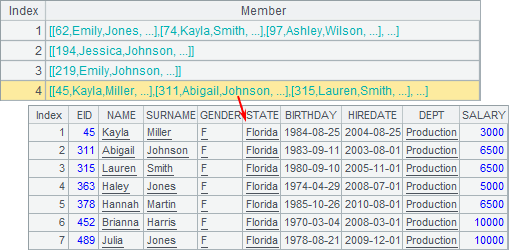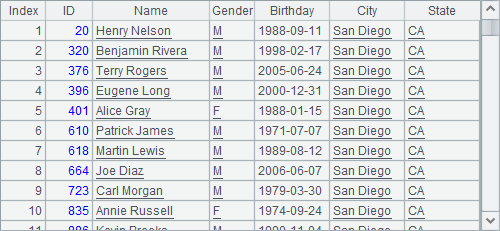A B C D 1 =file("D:/file/dw/students.ctx") =create(ID,City,School, SID) 2 for 20 ="C"/A2 =20+rand(31) 3 for C2 =B2/"_S"/B3 =rand(4001)+1000 4 =k(A2,B3) 5 for D3 =k(C5:2) 6 >B1.insert(0,C4+D5,B2, C3,D5) 7 =A1.create@y(#ID:4,City, School,SID;ID:2) >A7.append(B1.cursor()) =A1.open().import(;;10: 200) =A1.open().import(;;11: 200)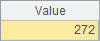A7中构建组表的基表，构建时使用了f.create(C:b, …; K:b) 函数，在排号数据构成的键排号键中指明了字节数，同时在后面的分段键中设置了分段时使用的字节数。在A7中，排号键ID4个字节，但在分段时只使用它的前2个字节。

C7中的数据如下：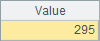D7中的数据如下：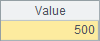A 1 =file("D:/file/dw/employees.ctx") 2 =A1.open() 3 =create(EID,Salary).keys(EID).record([1,10000,147,12000,179,9800]) 4 =A2.new(A3, EID, Dept, Name, Salary) 5 =A2.derive(A3.cursor(), Dept, Name; Dept=="Sales").fetch()

A3中新建了一个序表，包括主键EID和工资Salary两个字段，并插入了3条记录：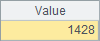A4中使用T.new(A/cs, x:C, …:w)函数，取出EIDDeptNameSalary4个字段构成新序表返回，其中DeptName是员工组表中的数据。在函数计算时，会根据A3中序表的主键，在组表的实表中查询与之匹配的记录读取所需字段的值。注意序表相对于实表的主键必须是有序的。A4中结果如下：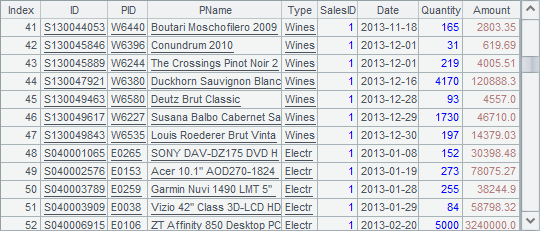A5中使用T.derive(A/cs, x:C, …:w)函数，取出组表的实表中的数据用来添加字段。这里使用游标计算，则结果也会返回游标，需要用fetch取出结果，另外函数中还添加了过滤条件，只取出销售部的员工数据，结果如下：